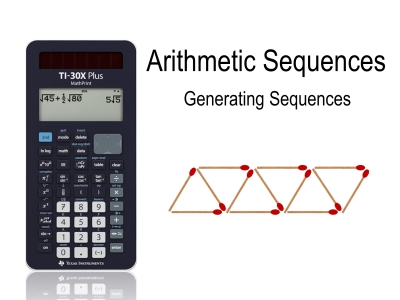# Activities

•• ##### AuthorAust Senior

45 Minutes

## Arithmetic Sequences#### Activity Overview

Students explore three different ways to generate a sequence on their calculator: recursively, using the sequence command (lists) and finally using the function editor. The memory locations a and d work nicely with the formula for an arithmetic sequence and make for a more dynamic and user-friendly environment.

This activity includes a video tutorial.

#### Objectives

The aim of this activity is to help students understand arithmetic sequences and identify effective and efficient techniques they can use on their TI-30XPlus MathPrint calculator.

#### Vocabulary

• Arithmetic Sequence
• Term
• Term Number
• Recursion
• Function
• Table